Welcome to Study Material Solution
Leave Comment

### UNIT

Measurement of any physical quantity is expressed in terms of an internationally accepted certain basic standard called unit.
Length, time & mass are measured in metre, sec, and kilogram

### PHYSICAL QUANTITIES

The quantities which can be measured by an instrument and by means of which we can describe the laws of physics are called physical quantities.
Eg.    length, velocity, acceleration, force, time, pressure, mass, density etc.

### REST & MOTION

An object is said to be at rest if it does not change its position with respect to its surrounding with the passage of time.
A body is said to be in motion if its position changes continuously with respect to the surroundings (or with respect to an observer) with the passage of time.

### UNIFORM & NON UNIFORM MOTION

(a) Uniform Motion :
A body has a uniform motion if it travels equal distances in equal intervals of time, no matter how small these time intervals may be.
For example, a car running at a constant speed of say, 10 m/s, will cover equal distances of 10 metres, in every second, so its motion will be uniform.

(b) Non-Uniform motion :
A body has a non-uniform motion if it travels unequal distance in equal intervals of time.
For example, if we drop a ball from the roof of a building, we will find that it covers unequal distances in equal intervals of time.
It covers :
4.9 meters in the 1st second
14.7 metres in the 2nd second
24.5 metres in the 3rd second  and so on.

### (a) Distance & displacement :

(i)  Distance : Distance is the actual path travelled by a body in a given time.
Consider a body travelling from A to B along any path between A and B. The actual length of the path that a body travels between
A and B is known as the distance. Here if the body goes from A to B via C, the distance travel will be ACB.
The distance travelled will be different for different paths between A and B. It is a scalar quantity.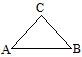(ii)  Displacement : The distance travelled in a given direction is the displacement. Thus displacement is the shortest distance between the given points.
S.I. unit of distance and displacement is metre.
Ex. When an object moves from O to B and then from B to A. Then displacement = x2 – x1.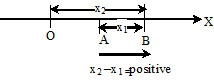Ex.     Suresh walks from point A to B and then again from B to C.Distance he has travelled is A to B + B to C.
Displacement he has travelled is line A to C

Note : If a body travels in such a way that it comes back to its starting position, then the displacement is zero.
However distance travelled never zero.

### (b) SPEED :

(i) Speed : Speed of a body is the distance travelled by the body in one second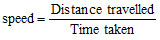Distance travelled is measured in metre and time in second.
Therefore, the unit of speed is metre/second.[(m/s)].
It can also be expressed in kilometer/hour [km/h]
If we know the speed of an object we can find out the distance covered by it in a given time. Distance covered= speed × time.
(ii) Average speed :
The speed of a bus during a journey may vary. When the bus is nearing a bus stop, its speed decreases. On the highways the
bus travels with greater speed but in a city or town it travels with less speed due to heavy traffic.
The bus has different speeds at different times. So we say that it has variable speed.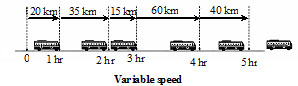For such bodies, we can calculate the average speed.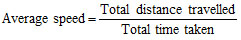If a body moves with the same speed at all times we say that it has uniform speed.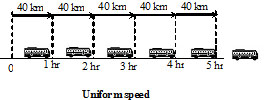### MEASUREMENTS

(a)  Measurement of time :
Many events in nature repeat themselves after definite intervals of time. For example, we can find that the sun rises everyday in the morning.
The time between one sunrise and the next was called a day. Similarly, a month was measured from one new moon to the next.
A year was fixed as the time taken by the earth to complete one revolution of the sun clocks or watches are perhaps
the most common time measuring devices.
All of them make use of some periodic motion. One of the most well-known periodic motions is that of a simple pendulum .
A simple pendulum consists of a small metallic ball or a piece of stone suspended from a rigid stand by a thread.
The metallic ball is called the bob of the pendulum.
Initially the pendulum at rest in its mean position. When the bob of the pendulum is released after taking it slightly to one side,
it begins to move to and fro. The to and fro motion of a simple pendulum is an example of a periodic or an oscillatory motion.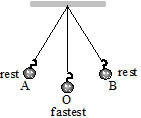The pendulum is said to have completed one oscillation when its bob, starting from its mean position O, moves  to A, to B and back to O.
The time taken by the pendulum to complete one oscillation is called its time period.
To set the pendulum in motion, gently hold the bob and move it slightly to one side. Now release the bob from its displaced position.
Measure the time the pendulum takes to complete 20 oscillations. Repeat this activity a few times and record your observations.
You will note that a slightly change in the initial displacement  not affect the time period of your pendulum.

Unit of time :
The basic unit of time is a second. Its symbol is s. Larger units of time are minutes (min) and hours (h).
Many time measuring devices were used in different parts of the world before the pendulum clocks became popular.
Sundials, water clocks and sand clocks are some examples of such devices.

(b) Measurement of speed : By calculating the distance travelled in the given time, we can calculate speed. Speed generally measured in m/s.
In vehicles speed is measured in km/h by the instrument speedometer.
(c)  Odometer : It measure the distance moved by vehicles directly.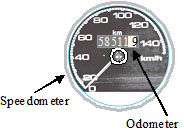### DISTANCE-TIME GRAPH

Consider the example : Rajesh was travelling with his father in their car from Kota to Bundi.  He kept himself busy by noting the distance travelled
by the car every 5 minutes. This is what he noted in the first 30 minutes.

 S.No. Time in minutes Distance in km 1 0 0 2 5 5 3 10 10 4 15 15 5 20 20 6 25 25 7 30 30

You can make a graphical representation of his observations follow these simple steps.
Taking axes and scale : Take a graph sheet an draw two lines perpendicular to each other.
Mark the horizontal line as OX (x-axis) and the vertical line as OY(y-axis). Time is taken on the X-axis and distance on the Y-axis.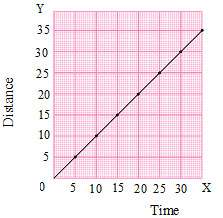Choose scales to represent distance and time.
For example, the scales could be
X-axis : 1 cm  =  5 minutes
Y-axis : 1 cm  =  5 km
Plotting the graph:  Mark the value on the axes for time and distance according to the scales you have chosen.
According to the values noted, mark the points on the graph sheet. Join the points
For uniform speed, the distance time graph is always a straight line.
For variable speed, it could be of any shape.

Ex.    Three cars A, B and C travel from Delhi to Agra. The time taken and the distance covered are given in the table below.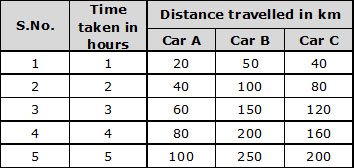Plot the distance time graph of the three cars in the same graph sheet.

Sol.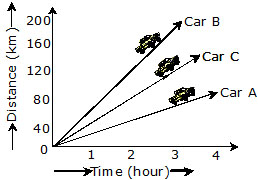Greater the speed, steeper will be the graph

### SOME IMPORTANT GRAPHS RELATED TO MOTION

All the following graphs are drawn for one-dimensional motion with uniform velocity or with constant acceleration.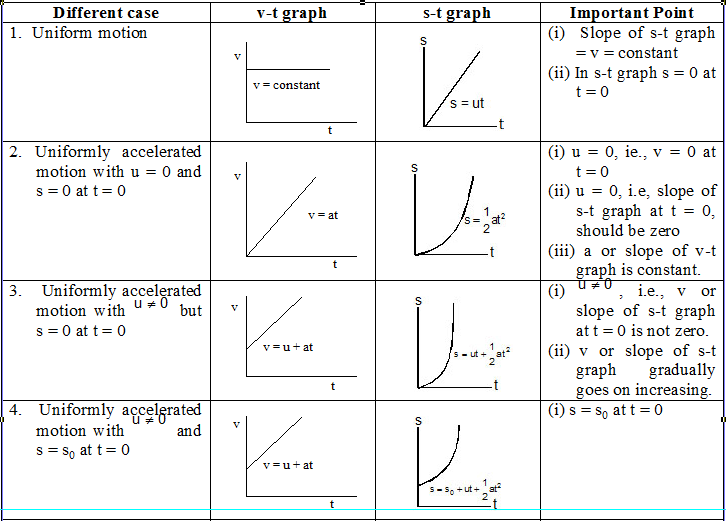### IMPORTANT POINTS

(1)    In case of motion under gravity for a given body, mass, acceleration, and mechanical energy remain constant while speed,
velocity, momentum, kinetic energy and potential energy change.
(2)    The motion is independent of the mass of the body, as in any equation of motion, mass is not involved.
This is why a heavy and lighter body when released from the same height, reach the ground simultaneously and with same velocity.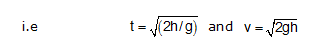However, momentum, kinetic energy or potential energy depend on the mass of the body (all  mass)
(3)    As from equation (2) time taken to reach a height h,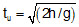Similarly, time taken to fall down through a distance h,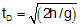so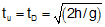So in case of motion under gravity time taken to go up a height h is equal to the time taken to fall down through the same height h.
(4)    If a body is projectd vertically up and it reaches a height h, then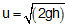and if a body falls freely through a height h, then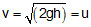So in case of motion under gravity, the speed with which a body is projected up is equal to the speed with which it comes back to the point of projection.

Q.1    Reena goes for a morning walk in the park near her house. She starts from point ‘A’ walks a circular path or radius 7 meter and returns to same point ‘A’.
(i) What is her displacement
(ii) Find the distance she has walked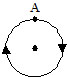Q.2    Classify the following as motion along a straight line, circular or oscillatory motion:
(i) Motion of your hands while running.
(ii) Motion of a horse pulling a cart on a straight road.
(iii) Motion of a child in a merry-go-round.
(iv) Motion of a child on a see-saw.
(v) Motion of the hammer of an electric bell.
(vi) Motion of a train on a straight bridge.

Q.3    A simple pendulum takes 32 s to complete
20 oscillations. What is the time period of the pendulum?

Q.4    The distance between two stations is 240 km. A train takes 4 hours to cover this distance. Calculate the speed of the train.

Q.5    Ram and Shyam walk from their home to the market in 20 minutes, Ram takes path 1 while Shyam takes path 2.
(i) What do you infer about their speeds ?
(ii) Who has the greater velocity? Why ?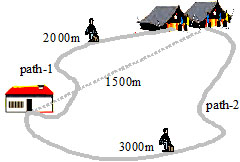Q.6    Which of the following are not correct?
(i)  The basic unit of time is second.
(ii)  Every object moves with a constant speed.
(iii) Distances between two cities are measured in kilometers.
(iv) The time period of a given pendulum is not constant.
(v)  The speed of a train is expressed in m/h.

Q.7     The odometer of a car reads 57321.0 km when the clock shows the time 08:30 AM. What is the distance moved by the car,
if at 08:50 AM, the odometer reading has changed to 57336.0 km? Calculate the speed of the car in km/min during this time.
Express the speed in km/h also.

Q.8    Meena takes 15 minutes from her house to reach her school on a bicycle. If the bicycle has a speed of 2 m/s,
calculate the distance between her house and the school.

Q.9    Show the shape of the distance-time graph for the motion in the following cases:
(i) A car moving with a constant speed.
(ii) A car parked on a side road.

Q.10    Which of the following relations is correct?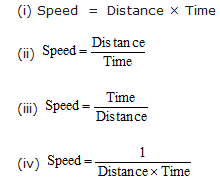(i) Speed  =  Distance × Time
(ii)
(iii)
(iv)

Q.11    A car moves with a speed of 40 km/h for 15 minutes and then with a speed of 60 km/h for the next 15 minutes.
The total distance covered by the car is:
(i) 100 km         (ii) 25 km
(iii) 15 km         (iv) 10 km

Q.12    Harish is travelling in a train moving at a speed of 72 km/h. In order to stop the train, the driver decreases the speed.
The rate of decrease in speed of the moving body is known as deceleration.
If the declaration of the train is 10m/s2, how much time will it take to come to a stop?

Q.13    Suppose the two photographs, shown in
figure 1 and figure 2 had been taken at an interval of 50 seconds. If a distance of 100 metres is shown by
1 cm in these photographs, calculate the speed of the blue car.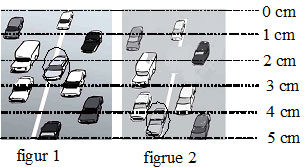Q.14    Figure shows the distance-time graph for the motion of two vehicles A and B. Which one of them is moving faster?Q.15    Which of the following distance-time graphs shows a truck moving with speed which is not constant?Q.16     The given graph depicts the motion of a bus. Interpret the motion of the bus.
(a) AB represents ............
(b) BC represents ............
(c) CD represents ............Q.1    A boy whose position with respect to surrounding does not change, is said to be in a state of-
(A) Rest        (B) Motion
(C) Vibration        (D) Oscillation

Q.2    In case of a moving body-
(A) Displacement  > Distance
(B) Displacement < Distance
(C) Displacement ³ Distance
(D) Displacement £ Distance

Q.3    A distance is always -
(A) Shortest length between two points
(B) path covered by and object between two points
(C) product of length and time
(D) none of these

Q.4    Which of the following is not characteristic of displacement ?
(A) It is always positive
(B) It has both magnitude and direction
(C) It can be zero
(D) Its magnitude is less than or equal to the actual path length of the object

Q.5    In five minutes distance between a pole and a car changes progressively. What is true about the car ?
(A) Car is at rest
(B) Car is in motion
(C) Nothing can be said with this information
(D) None of the above

Q.6    A distance-
(A) Is always positive
(B) Is always negative
(C) May be positive as well as negative
(D) Is neither positive nor negative

Q.7    A particle is travelling with a constant speed. This means-
(A)  Its position remains constant as time passes
(B)  It covers equal distance in equal interval of time
(C)  Its acceleration is zero
(D) It does not change its direction of motion

Q.8    In 10 minutes, a car with speed of 60 kmh–1 travels a distance of
(A) 6 km          (B) 600 km
(C) 10 km        (D) 7km

Q.9    A car acceleration uniformaly from 18 km/h to 36 km/h in 5s. The acceleration in ms–2 is-
(A) 1        (B) 2
(C) 3        (D) 4

Q.10    The brakes applied to a car produce a negative acceleration of 6ms–2.
If the car stops after 2 seconds, the initial velocity of the car is-
(A) 6ms–1           (B) 12 ms–1
(C) 24 ms–1        (D) Zero

Q.11    A body is moving with uniform velocity of
10 ms–1. The velocity of the body after 10 s is-
(A) 100 ms–1        (B) 50 ms–1
(C) 10 ms–1          (D) 5 ms–1

Q.12    In 12 minutes a car whose speed is 35 kmh–1 travels a distance of-
(A) 7 km          (B) 3.5 km
(C) 14 km        (D) 28 km

Q.13    A body is moving along a straight line at20 ms–1  undergoes an
acceleration of 4ms–2. After 2 s. its speed will be-
(A) 8 ms–1          (B) 12 ms–1
(C) 16 ms–1        (D) 28 ms–1

Q.14    A car increases its speed from 36 km/h to
54 km/h in 10s. Its acceleration is-
(A) 30 ms–2        (B) 3 ms–2
(C) 18 ms–2        (D) 0.5 ms–2

Q.15    The device used to measure speed of a vehicle is -
(A) Odometer              (B) Speedometer
(C) Thermometer        (D) Voltmeter

Q.16   The duration of the day from the moment the sun is over head today to the moment the
Sun is overhead tomorrow is determined by-
(A)  the rotation of Earth around the sun
(B)  the revolution of Earth on its axis
(C)  the inclination of the axis of rotation of the Earth from its plane of revolution
(D)  The rotation and revolution of Earth around the sun.

Q.17    The increase in the speed of a car is proportional to the additional petrol put into the engine.
Is it possible to accelerate a car without putting more petrol or less petrol into the engine ?
(B)  Not possible, the statement is wrong
(C) May be possible if there is a leakage in the car
(D) Yes it is possible provided the car moves in circular path.

1.       A             2.      D             3.     B             4.     A
5.       B            6.      A               7.     B            8.     C
9.       A            10.     B             11.    C           12.    A
13.     D           14.      D           15.     B          16.     B
17.     D

### NTSE/MAINS PROBLEMS

Q.1.    A runner completes one round of a circular path of radius r in 40 seconds. His displacement after 2 minutes 20 seconds will beQ.2.    An old man goes for morning walk on a semicircular track of radius 40 m; if he starts from one end of the track and reaches
to other end, the distance covered by the man and his displacement will respectively be
 126 m, 80 m     80 m, 126 m     80 m, 252 m     252 m, 80 m

Q.3.    A body covered a distance of L m along a curved path of a quarter circle.  The ratio of distance to displacement isQ.4.    A passenger travels along a straight line with velocity v1 for first half time and with velocity
v2 for next half time, then the mean velocity v is given byQ.5.    A car covers a distance of 2 km in 2.5 minute, if it covers half of the distance with speed 40 km/hr, the rest distance it will cover with speed

 56 km/hr     60 km/hr     50 km/hr     48 km/hr

Q.6.    A bicyclist encounters a series of hills.  Uphill speed is always v1 and downhill speed is always v2.  The total distance travelled is ,
with uphill and downhill portions of equal length.  The cyclist's average speed isQ.7.    A motor car covers rd part of total distance with , second rd part with  km/hr and rest rd part with .  What is the average speed of the car?
 18 km/hr     45 km/hr     6 km/hr     22.5 km/hr

Q.8.    The displacement-time graph for the two particles A and B are straight lines inclined at angles 300 and 600 with the time axis.
The ratio of the velocities of A to B will be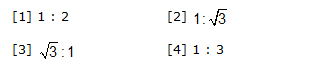Q.9.    The velocity-time graph of a body is shown in figure.  The displacement covered by the ody in 8 seconds is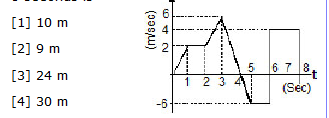Q.10.  The variation of velocity of a particle moving along straight line is shown in figure.
The distance traversed by the body in 4 seconds is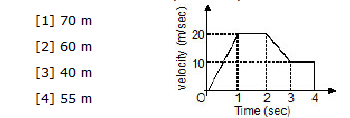Q.11. The displacement y (in metres) of a body varies with time (in seconds) according to the equation y = -2/3 t+16t+2

How long does the body come to rest?
 8 seconds     10 seconds     12 seconds     14 seconds

Q.12. If y denotes the displacement and t denotes the time and the displacement is given by , the velocity of the particle is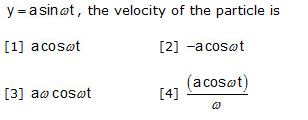Q.13.A truck travelling due to North at 20 m/s turns East and travels at the same speed.  The change in its velocity is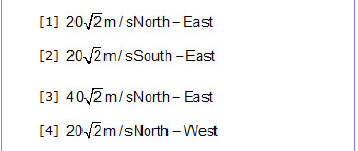Q.14.   Which one of the following equations represent the motion of a body with finite constant acceleration.
In these equations y denotes the displacement of the body at time t and a,b and c are the constant of the motion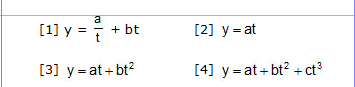Q.15.    A car moving at 12 m/s due east attains a speed 16 m/s towards north in 4 seconds.
The magnitude of its average acceleration in m/s2 is
 1 m/s2     3 m/s2     5 m/s2     7 m/s2

Q.16.   Adjacent graph shows the variation of velocity of a rocket with time.
Find the time of burning of fuel from the graph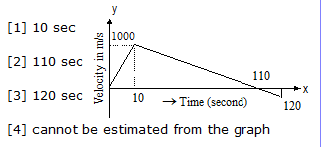Q.17.   The adjoining curve represents the velocity-time graph of a particle, its acceleration
values along OA, AB and BC in metre/sec2 are respectivelyQ.18.   A car travels first 1/3 of the distance AB at 30 km/hr next 1/3 of the distance at 40 km/hr, last 1/3
of the distance at 24 km/hr.  Its average speed in km/hr for the whole jouney is
 40     35     30     28

Q.19.   A particle travels A to M along a straight line with a velocity of 8 m/s and M to A with a velocity
of 2 m/s, then the average velocity for the whole journey is
 3.2 m/s     –5 m/s     –3.2 m/s     0 m/s

Q.20.  An object will continue accelerating untill
 the resultant force on it begins to decrease
 the velocity changes direction
 the resultant force on it is zero
 the resultant force is at right angles to its direction of motion

Q.21.  A girl walks along an east-west street, and a graph of her displacement from home is as shown in figure.
Her average velocity for the whole time intervals is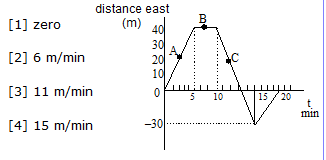Q.22.  The uniform motion in the following acceleration time graph is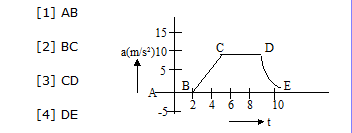AB
 BC
 CD
 DE

Q.23.  The displacement-time relationship for a particle is given by x = a0+a1t+t+a2t2.
The acceleration of the particle is
               

Q.24.   A particle travels for 40 seconds under the influence of a constant force.
If the distance travelled by the particle is S1 in the first twenty seconds and
S2 in the next twenty seconds, then
 S2 = S1     S2 = 2S1     S2 = 3S1     S2 = 4S1

Q.25.  A particle moves with constant acceleration for 6 seconds after starting from rest.
The distance travelled during the consecutive 2 seconds interval are in the ratio
 1 : 1 : 1     1 : 2 : 3     1 : 3 : 5     1 : 5 : 9

Q.26.  A body having initial velocity u is moving with uniform acceleration a.
The distance covered by it in nth second is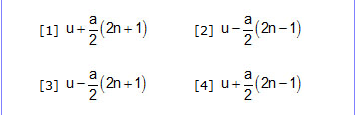Q.27.  A ball is thrown upward and reaches a height of 64 feet, its initial velocity should be (g = 32 ft/sec2)

 64 ft/sec     72 ft/sec     32 ft/sec     4096 ft/sec

Q.28.   Two bodies of different masses ma and mb are dropped from two different heights, viz a and b.
The ratio of times taken by the two to drop through these distance is

 a : b              

Q.29.  A stone weighing 10 kg is dropped from a cliff in a high wind.The wind exerts a steady horizontal
force of 50 N on the stone as it falls.The path that the stone follows will be

 A parabola                            An ellipse
 A more complicated path     A straight line

Q.30.   A body thrown up with a finite speed is caught back after 4 sec.
The speed of the body with which it is thrown up is

 10 m/sec     20 m/sec     30 m/sec     40 m/sec

Q.31.  A ball is dropped from certain height on a glass floor so that it rebounds elastically to the same height.
If the process continues, the velocity-time graph for such a motion would beQ.32.  A stone is thrown vertically upwards with an initial velocity of 30 m/s.
The time taken for the stone to rise to its maximum height is
 0.326 s     3.26 s     30.6 s     3.06 s

Q.33. A body is thrown upward and reaches its maximum height.  At that position
 its velocity is zero and its acceleration is also zero
 its velocity is zero but its acceleration is maximum
 its acceleration is minimum
 its velocity is zero and its acceleration is the acceleration due to gravity

Q.34. The initial velocity of a particle (at t = 0) is u and the acceleration of particle at time t is given by f = at.
Where a is a constant which of the following relation for velocity v of particle after time t is true?
 v = u + at2     v = u + at2/2     v = u + at     none of these

Q.35. A man is walking on a road with a velocity 3 km/hr.  Suddenly rain starts falling.
The velocity of rain is 10 km/hr in vertically downward direction.  The relative velocity of the rain is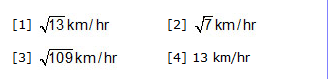Q.36. A boat P is moving at 40 km/hr and another boat Q is moving at 20 km/hr.  Which one of the following
is not a possible value for their relative velocity

 10 km/hr     20 km/hr     30 km/hr     40 km/hr

Q.37.    The position of a body with respect to time is given by x = 4t3 – 6t2 + 20 t + 12.  Acceleration at t = 0 will be

 –12 units     12 units     24 units     –24 units

Q.38.   A body travels 200 cm in the first two seconds and 220 cm in the next four second.
The velocity at the end of the seventh second from the start will be

 10 cm/s     5 cm/s     15 cm/s     20 cm/s

Q.39.  A man standing on a road hold his umbrella at 300 with the vertical to keep the rain away.
He throws the umbrella and starts running at 10 km/h.  He finds that raindrops are hitting his head vertically,
the speed of rain drop with respect to the road will be

 10 km/h     20 km/h     30 km/h     40 km/h

Q.40.  The linear momentum of a body is p.  The linear momentum p varies with time.
The equation for variation is p = a + bt2 where a and b are constants.
The effective force acting on the body is

 proportional to t2
 constant
 proportional to t
 inversely proportional to t

Q.41.  The acceleration of a particle increases linearly as bt with time.
If the particle starts from v0 as initial velocity then the distance travelled in t seconds will be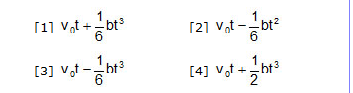Q.42. A particle has velocity given by v = 20 + 0.1 t2 then it has
 uniform acceleration             uniform retardation
 non uniform acceleration     zero acceleration

Q.43.  A body starting from rest and has uniform acceleration 8 m/sec2.
The distance travelled by it in 5th second will be

 36 m     40 m     100 m     200 m

Q.44.  A body starts from rest, the ratio of distances travelled by the body during 3rd and 4th seconds is

 7/5     5/7     7/3     3/7

Q.45.  A body sliding on a smooth inclined plane requires 4 sec to reach the bottom after starting from rest at the top.
How much time does it take to cover one fourth the distance starting from the top

 1 sec     2 sec     0.4 sec     1.6 sec

Q.46.  The initial velocity of a particle is 10 m/sec and its retardation is 2 m/sec2.
The distance covered in the fifth second of the motion will be

 1 m     19 m     50 m     75 m

Q.47.  A particle is moving east-wards with a velocity of 5m/sec.  In 10 seconds its velocity changes to 5m/sec north-wards.
The average acceleration during this time is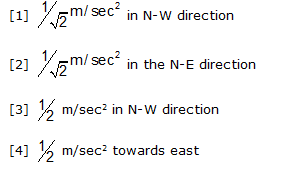Q.48.  A ship of mass 3 x 107 kg initially at rest, is pulled by a force of 5 x 104 N through a distance of 3m.
Assuming that the resistance due to water is negligible, the speed of the ship is

 1.5 m/sec     60 m/sec     0.1 m/sec     5 m/sec

Q.49. A particle moves with a constant acceleration such that in the successive time intervals t1 ,
t2 and t3 its average velocities are v1, v2 and v3.  The ratio of v1 – v2 and v2 – v3 is

                t1 – t2 : t2 – t3

Q.50.    A car travels from place A to the place B at 20 km/hour and returns at 30 km/hour.
The average speed of the car for the whole journey is

 25 km/hour     24 km/hour     50 km/hour     5 km/hour### EXERCISE-I

Section A
•    Fill in the blanks
1.    Soldiers in a march part moves __________.
2.    Motion of the earth around the sun _________.
3.    Motion of a pendulum __________
4.    The distance covered by an object in a unit time is called ________.
5.    The time taken by the pendulum to complete one oscillaution is called its ________.
6.    In an electronic watch, the component corresponding to the pendulum of a pendulum clock is a ________
7.    In the given graph, increase in speed with increase in time is ________.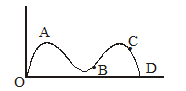8.    In the following distance time graph showing the motion of two vehicles A and B, _______ is moving faster than other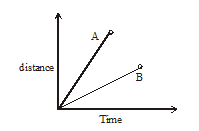9.    Which of the following distance-time graphs shows a truck moving with speed which is not constant?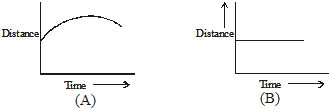### Section B

•    True or false
1.    Hands of an athlete in a race moves along a straight line.
2.    Motion of a swing in periodic motion.
3.    Faster vehicle has a slower speed.
4.    The to and fro motion of a simple pendulum is an example of a oscillatory motion.
5.    the symbols of all units are written is singular.
6.    The heart-beats about-12 times in 10 second.
7.    The smallest time interval that-can be measured with commonly available clocks is one second.
8.    Motion of objects can be presented in pictorial form by their distance time graphs.
9.    The distance-time graph for the motion of an object moving with a variable speed is a straight time.
10.   The average distance between Earth and the sun is called one astronomical unit (A.U.).

( 1 marks)
1.    What is an oliscillatroy motion ?
2.    Define Time period.
3.    Write the relation between distance covered, speed and time.
4.    Name the meter, which is used to records speed directly.
5.    Name the meter, which measures the distance moved by the vehicle.
6.    Write the basic unit of speed.
7.    The most accurate clock in the world is developed by the institute (name)
8.    How much time the most accurate watch lose or gain after running for 20 million years.
9.    Define Time.
10.    What is solar day ?

(2 marks)
1.    What are quartz clocks ?
2.    What is BAR graph ?
3.    What do you mean by uniform and non-uniform motion ?
4.    Explain the following terms.
(a) Reference point                (b) Stop watch
(c) Sundial                             (d) Digital clock
5.    Give the equivalenst unit of the following
(a) Ten year                   (b) Hundred year
(c) 1000 year

(3 marks)
1.    With the help of diagram of simple perpendicular explain time period, amplitude and frequency.
2.    The motion of a car is given below.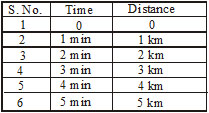Plot the graph and conclude the nature of motion.
3.    What is gnomon ? Explain.
4.    A pendulum takes 25 sec to complete 20 oscillations. What is the time period of the pendulum. (1.25 sec)
5.    The distance between Amritsar and Delhi is 450 km. If train takes 6 h to cover this distance,
what is the average speed of the train ?    (75 km/h)

(4 marks)
1.    A bus moves at a constant speed of 45 km/h for 10 minutres. What is teh distance covered by the bus?
2.    In how much time will a train moving at 90 km/h cover a distance of 15 km ?
3.    A body is moving along a circular path of radius 7 m. What will be the distance covered by the body, when it completes half a revolution.
4.    Plot distance time graph, when
(i) Body is moving with uniform speed.        (ii) Body is at rest.
5.    A car covers a distance of 5 km in 10 minutes. Find the speed in
(i) km/min     (ii) m/sec    (iii) m/min    (iv) km/h

EXERCISE-III

SECTION-A
•      MCQ with one choice correct
1.    Which one is correct
(A) Speed = Distance Time               (B) Speed = Distance / Time
(C) Speed = Time / Distance             (D) Speed =  Time

2.  The basic unit of speed is
(A) km/min        (B) m/min         (C) km/h        (D) m/sec

3.   A car moves with a speed of 40 km/h for 15 minutes and then with a speed of
60 km/h for the next 15 min. The total distance convered by the car is :
(A) 100 km         (B) 25 km         (C) 15 km        (D) 100 km ]

4.  A car moves 1 hour with an speed of 10 km/h. How much distance it will cover in 2 hours.
(A) 10 km        (B) 20 km         (C) 30 km         (D) 40 km

5.  A girl runs a distance of 160 m in 20 sec. What is the speed of girl.
(A) 10 m/sec        (B) 8 m/sec        (C) 12 m/sec        (D) 14 m/sec

6.  One solar day is equal to
(A) 90,000 sec    (B) 86,400 sec    (C) 80,000 sec    (D) 95,000 sec

7.   A device in which falling sand is used as timing device in called
(A) Watch         (B) Speed         (C) Sand clock     (D) None of these

8.  The ratio between the distance covered and the time is called
(A) Speed         (B) Frequency         (C) Time period     (D) Amplitude

9.  The to and fro motion of a pendulum about its mean pontion is called.
(A) Oscillation         (B) Frequency         (C) Time period    (D) Amplitude

10.  One thousand years is equal to
(A) Decade         (B) Century         (C) Millennium         (D) None of these

SECTION-B
•    NTSE Competition Based
1.  What is machanics ?
2.  What is statics ?
3.  What is the kinematics ?
4.  What is Dynamics ?
5.  What do you mean by Absolute motion ?
6.  Can distance be negative ?
7.  Can speed be negative ?
8.  A body cover distances x1, x2, x3... in the time intervals t1, t2, t3.... then find the average speed of the body.
9.  A body travels a distance of 10 m towards south, then he runs towards east and travels
12 m is that direction. Fidn the distance travelled.
10. A train covers half of its journey with a speed of 60 m/s and other half with a speed of 40 m/s.
Calculate the average speed of the train during the whole journey.

Exercise-I

SECTION-A
1.Along a Straight line            2. Circular                  3. Periodic
4. Speed                                   5. Time period           6. Quratz crystal oscillutor
7. OA                                        8. A                            9. A

SECTION-B
1.    (F)    2.    (T)    3.    (F)    4.      (T)    5.    (T)     6.    (T)
7.    (T)    8.    (T)    9.    (F)    10.    (T)

Exercise-III

SECTION-A
1.    (A)     2.    (D)     3.    (*)    4.        (*)    5.    (B)     6.    (B)
7.    (C)     8.    (A)    9.    (A)     10.    (C)